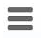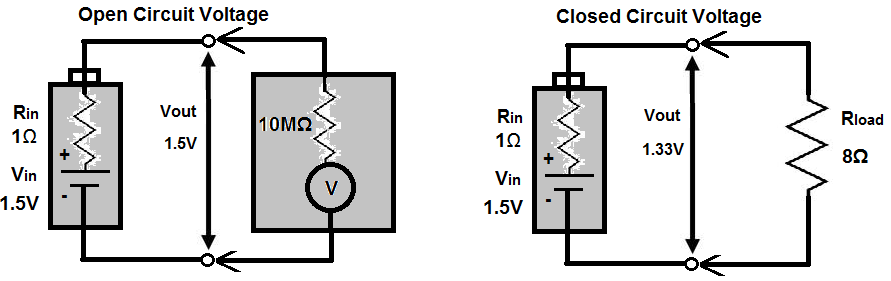﻿ Battery Internal Resistance# Battery Internal ResistanceAll batteries have some internal resistance to some degree.

Batteries have internal resistance because the elements that make it up aren't perfect conductors. The electrodes and electrolytes aren't 100% conductive. So they will have some resistance (internal resistance) in them.

Ideally, a battery should have 0Ω internal resistance. So during battery operation, all the voltage will be dropped across the element that the battery is powering instead of the battery dropping voltage across itself. According to voltage division, voltage drops across the element with the higher impedance. Ohm's law tells you this in V=IR, showing the higher the resistance, the greater the voltage drop. So if a battery has 0Ω of resistance and it will power a device that has at least some impedance, this ensures, according to ohm's law, that the device will get the voltage and not the battery. This is the ideal case but it doesn't occur in real life.

Batteries will always have some resistance. Though the internal resistance may be or appear low, around 0.1Ω for an AA alkaline battery, and about 1Ω to 2Ω for a 9-volt alkaline battery, it can cause a noticeable drop in output voltage if a low-resistance load is attached to it.

This is understood when you take into account how voltage division works. Taking into account ohm's law, voltage is equal to current * resistance (v=ir). The larger the resistance is, the more voltage gets allocated to that component. If a component has a very large impedance, that component will get most of the voltage, which comes from the battery. However, if the component has a small impedance, say, a few ohms, the voltage output from the battery will not necessarily be received fully by the component. Voltage may be lost, because the battery's internal resistance may compete with that element and the battery will drop voltage across itself instead of the component. This is for small load impedances. Remember, voltage gets allocated according to impedance values. So the impedance of the output device and the battery will always be divided up between each other.

Without any load, we can meaure the open-circuit voltage of the battery by placing a multimeter on the DC voltage setting to measure voltage. This voltage is essentially equal to the battery's rated nominal voltage- the voltmeter has such high input impedance that all of the voltage drops across it. Therefore, it's able to measure the voltage fully. Another way to consider it is that is has such high input impedance that it draws practically no current (v=ir), so there is no appreciable voltage drop.

However, if we attach a load to the battery, the output terminal voltage of the battery drops. By treating the internal resistance Rin and the load resistance Rload as a voltage divider, you can calculate the true output voltage presence across the load.Batteries with large internal resistance show poor performance in supplying high current pulses. This is because current is decreased with higher resistance. Current equals voltage divided by resistance (i=v/r). So the higher the internal resistance, the lower the current output ability. Low internal resistance batteries are much better at supplying high current pulses.

Internal resistance also increases as the battery discharges. Therefore, a typical alkaline AA battery may start out with an internal resistance of 0.15Ω but may increase to 0.75Ω when 90 percent discharged.

The following is a list of typical internal resistances for various batteries. However, the values cannot be assumed to be universal. Check the specific specifications of your battery in use to find out the exact values.

 Battery Internal Resistance 9-V zinc carbon 35Ω 9-V lithium 16Ω to 18Ω 9-V alkaline 1Ω to 2Ω AA alkaline 0.15Ω AA NiMH 0.02Ω D Alkaline 0.1Ω D NiCad 0.009Ω D SLA 0.006Ω AC13 zinc-air 5Ω 76 silver 10Ω 675 mercury 10Ω

Both AA alkaline and AANiMH double in resistance after a 50 percent discharge.

This table is useful when selecting batteries. The lower the internal resistance, the more desirable the battery. The lower the internal resistance, the more current it can output. However, the batteries all have their different uses, and if high current output is not a necessity, other battery selections can be just as useful.

Related Resources